### Home > MC2 > Chapter 10 > Lesson 10.2.2 > Problem10-98

10-98.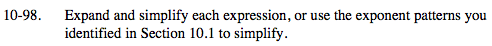Refer to the Math Notes box in Lesson 10.1.6 to review the Laws of Exponents.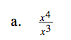When dividing like variables, subtract the exponent in the denominator from the exponent in the numerator.

x(4-3)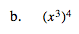When there is an exponent outside of the parentheses, multiply the exponent with the exponent of every variable inside the parentheses.

x(4)-(3)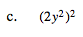22 y(2)(2)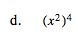See part (b).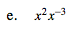Use the rule (xa)(xb) = x(a+b).

x-1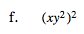See part (c).

x2y4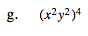See part (c).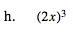See part (c).

8x3